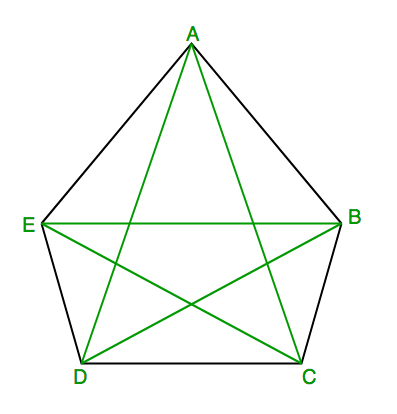# Find number of diagonals in n sided convex polygon

• Difficulty Level : Medium
• Last Updated : 22 Jun, 2022

Given n > 3, find number of diagonals in n sided convex polygon.
According to Wikipedia, In geometry, a diagonal is a line segment joining two vertices of a polygon or polyhedron, when those vertices are not on the same edge. Informally, any sloping line is called diagonal.

Examples :

```Input : 5
Output : 5```

Explanation: Five possible diagonals are : AC, AD, BD, BE, CERecommended Practice

Since for an n-sided convex polygon, from each vertex, we can draw n-3 diagonals leaving two adjacent vertices and itself. Following this way for n-vertices, there will be n*(n-3) diagonals but then we will be calculating each diagonal twice so total number of diagonals become n*(n-3)/2
Here is code for above formula.

## C++

 `#include ``using` `namespace` `std;` `// C++ function to find number of diagonals``// in n sided convex polygon``int` `numberOfDiagonals(``int` `n)``{``    ``return` `n * (n - 3) / 2;``}` `// driver code to test above function``int` `main()``{``    ``int` `n = 5;``    ``cout << n << ``" sided convex polygon have "``;``    ``cout << numberOfDiagonals(n) << ``" diagonals"``;``    ``return` `0;``}`

## Java

 `// Java function to find number of diagonals``// in n sided convex polygon` `public` `class` `Diagonals {` `    ``static` `int` `numberOfDiagonals(``int` `n)``    ``{``        ``return` `n * (n - ``3``) / ``2``;``    ``}` `    ``// driver code to test above function``    ``public` `static` `void` `main(String[] args)``    ``{``        ``int` `n = ``5``;``        ``System.out.print(n + ``" sided convex polygon have "``);``        ``System.out.println(numberOfDiagonals(n) + ``" diagonals"``);``    ``}``}` `// This code is contributed by Saket Kumar`

## Python3

 `# Python3 program to find number of diagonals``# in n sided convex polygon``def` `numberOfDiagonals(n):``    ``return` `n ``*` `(n ``-` `3``) ``/` `2` ` ` `# driver code to test above function``def` `main():``    ``n ``=` `5``    ``print``(n , ``" sided convex polygon have "``)``    ``print``(numberOfDiagonals(n) , ``" diagonals"``)` `if` `__name__ ``=``=` `'__main__'``:``    ``main()` `#this code contributed by 29AjayKumar`

## C#

 `// C# function to find number of diagonals``// in n sided convex polygon``using` `System;` `class` `GFG {``    ` `    ``static` `int` `numberOfDiagonals(``int` `n)``    ``{``        ``return` `n * (n - 3) / 2;``    ``}` `    ``// driver code to test above function``    ``public` `static` `void` `Main()``    ``{``        ``int` `n = 5;``        ``Console.Write(n + ``" sided convex polygon have "``);``        ` `        ``Console.WriteLine(numberOfDiagonals(n) +``                                  ``" diagonals"``);``    ``}``}` `// This code is contributed by Sam007`

## PHP

 ``

## Javascript

 ``

Output :

`5 sided convex polygon have 5 diagonals`

Time Complexity: O(1)

Auxiliary Space: O(1)

This article is contributed by Pratik Chhajer. If you like GeeksforGeeks and would like to contribute, you can also write an article using write.geeksforgeeks.org or mail your article to review-team@geeksforgeeks.org. See your article appearing on the GeeksforGeeks main page and help other Geeks.
Please write comments if you find anything incorrect, or you want to share more information about the topic discussed above.

My Personal Notes arrow_drop_up